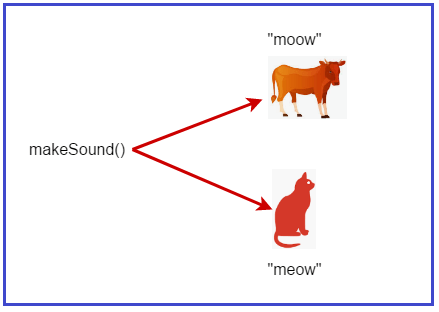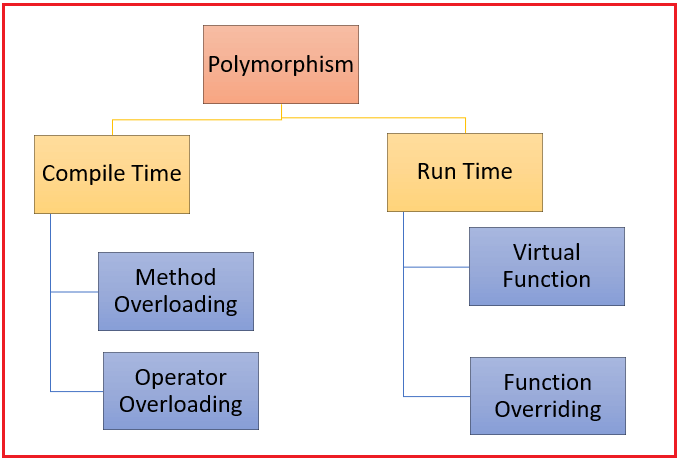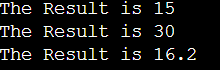# Polymorphism in C++

## Polymorphism in C++ with Examples

In this article, I am going to discuss Polymorphism in C++ with Examples. Please read our previous article where we discussed Base Class Pointer and Derived Class Object in C++ with Example.

##### What is Polymorphism in C++?

The word polymorphism means having many forms. In simple words, we can define polymorphism as the ability of a message to be displayed in more than one form. One real-life example of polymorphism is, that a person at the same time can have different characteristics. A man at the same time is a father, a husband, and an employee. So, the same person possesses different behavior in different situations. This is called polymorphism. Polymorphism is considered one of the important features of Object-Oriented Programming.

In C++, polymorphism causes a member function to behave differently based on the object that calls/invokes it. Polymorphism is a Greek word that means to have many forms. It occurs when you have a hierarchy of classes related through inheritance. For example, suppose we have the function makeSound(). When a cat calls this function, it will produce a meow sound. When a cow invokes the same function, it will provide the moow sound.Though we have one function, it behaves differently under different circumstances. The function has many forms; hence, we have achieved polymorphism.

##### Types of Polymorphism in C++:

C++ supports two types of polymorphism. They are as follows:

2. Runtime Polymorphism (Example: Function Overriding and Virtual Functions)

For a better understanding, please have a look at the below image.##### Compile Time Polymorphism in C++:

This type of polymorphism is achieved by function overloading or operator overloading. The overloaded functions are invoked by matching the type and number of arguments. The information is present during compile-time. This means the C++ compiler will select the right function at compile time. Compile-time polymorphism is achieved through function overloading and operator overloading which is also known as static binding or early binding.

When there are multiple functions with the same name but different parameters then these functions are said to be overloaded. Functions can be overloaded by changes in the number of arguments or/and changes in the type of arguments. That means function overloading in C++ occurs when we have many functions with similar names but different arguments. The arguments may differ in terms of number or type.

```#include <iostream>
using namespace std;

{
int sum = num1 + num2;
cout << "The Result is " << sum << endl;
}

void Add(int num1, int num2, int num3)
{
int sum = num1 + num2 + num3;
cout << "The Result is " << sum << endl;
}

{
double sum = num1 + num2;
cout << "The Result is " << sum << endl;
}

int main()
{
return 0;
}
```
###### Output:In the above example, a single function named Add acts differently in three different situations which is the property of polymorphism. Please click on the below link to learn about Function Overloading in detail.

C++ also provides the option to overload operators. In Operator Overloading, we define a new meaning for a C++ operator. It also changes how the operator works. For example, we can make the operator (+) for string class to concatenate two strings. We know that this is the addition operator whose task is to add two operands. So, a single operator “+” when placed between integer operands, adds them and when placed between string operands, concatenates them.

```#include<iostream>
using namespace std;

class ComplexNum
{
private:
int real, over;

public:
ComplexNum (int rl = 0, int ov = 0)
{
real = rl;
over = ov;
}

ComplexNum operator + (ComplexNum const &obj)
{
ComplexNum result;
result.real = real + obj.real;
result.over = over + obj.over;
return result;
}
void Print()
{
cout << real << " + i" << over << endl;
}
};

int main()
{
ComplexNum c1 (10, 2), c2 (3, 7);
ComplexNum c3 = c1 + c2;
c3.Print();
}
```

Output: 13 + i9

##### Runtime Polymorphism in C++:

Run time polymorphism is achieved when the object’s method is invoked at the run time instead of compile time. It is achieved by method overriding which is also known as dynamic binding or late binding.

This type of polymorphism is achieved by Function Overriding. This happens when an object’s method is invoked/called during runtime rather than during compile time. Runtime polymorphism is achieved through function overriding. The function to be called/invoked is established during runtime.

##### Function Overriding in C++:

Function overriding on the other hand occurs when a derived class has a definition for one of the member functions of the base class. That base function is said to be overridden. Function overriding occurs when a function of the base class is given a new definition in a derived class. At that time, we can say the base function has been overridden.

```#include <iostream>
using namespace std;
class Base
{
public:
void Display()
{
cout << "Base Class Display Function";
}
};

class Derived:public Base
{
public:
void Display()
{
cout << "Derived Class Display Function";
}
};

int main()
{
Derived d;
d.Display();
return 0;
}
```

Output: Derived Class Display Function

Function Overriding in C++ with Examples

##### Virtual Function in C++:

A virtual function is another way of implementing run-time polymorphism in C++. It is a special function defined in a base class and redefined in the derived class. To declare a virtual function, you should use the virtual keyword. The keyword should precede the declaration of the function in the base class. If a virtual function class is inherited, the virtual class redefines the virtual function to suit its needs.

```#include <iostream>
using namespace std;
class Base
{
public:
void virtual Display()
{
cout << "Base Class Display Function";
}
};

class Derived:public Base
{
public:
void Display()
{
cout << "Derived Class Display Function";
}
};

int main()
{
Base *p = new Derived();
p->Display();
return 0;
}
```

Output: Derived Class Display Function

Virtual Functions in C++ with Examples

##### Compile-Time Polymorphism Vs. Run-Time Polymorphism in C++:
###### Compile-Time Polymorphism in C++:
1. The function to be invoked is known at the compile time.
2. It’s also called early binding or static polymorphism.
3. The method is called/invoked during compile time.
5. Faster execution since the discovery of the method is done during compile time.
6. Less flexibility for problem-solving is provided since everything is known during compile time.
7. Overloading is a compile-time polymorphism where more than one method is having the same name but with a different number of parameters or the type of the parameters.
###### Run-Time Polymorphism in C++:
1. The function to be invoked is known at the run time.
2. It’s also called late/dynamic binding or dynamic polymorphism.
3. The method is called/invoked during run time.
4. It is Implemented via method overriding and virtual functions.
5. Slower execution since method discoverer is done during runtime.
6. Much flexibility is provided for solving complex problems since methods are discovered during runtime.
7. Overriding is a run time polymorphism where more than one method is having the same name, number of parameters, and the type of the parameters.
##### Why Polymorphism in C++?

Polymorphism allows us to create consistent code. For example, suppose we need to calculate the area of a circle and a square. To do so, we can create a Shape class and derive two classes Circle and Square from it. In this case, it makes sense to create a function having the same name calculateArea() in both the derived classes rather than creating functions with different names, thus making our code more consistent.

Note: The term “Polymorphism” is the combination of “poly” + “morphs” which means many forms. It is a greek word. In object-oriented programming, we use 3 main concepts: inheritance, encapsulation, and polymorphism. Typically, polymorphism occurs when there is a hierarchy of classes and they are related by inheritance. C++ polymorphism means that a call to a member function will cause a different function to be executed depending on the type of object that invokes the function.